Next: Mass Conservation Up: Mathematical Models of Fluid Previous: Viscosity

# Conservation Laws

Suppose that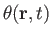is the density of some bulk fluid property (e.g., mass, momentum, or energy) at position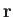and time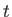. In other words, suppose that, at time, an infinitesimal fluid element of volume, located at position, contains an amount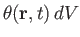of the property in question. Note, incidentally, thatcan be either a scalar, a component of a vector, or even a component of a tensor. The total amount of the property contained within some fixed volumeis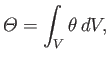(1.28)

where the integral is taken over all elements of. Let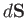be an outward directed element of the bounding surface of. Suppose that this element is located at point. The volume of fluid that flows per second across the element, and so out of, is. Thus, the amount of the fluid property under consideration that is convected across the element per second is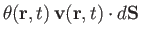. It follows that the net amount of the property that is convected out of volumeby fluid flow across its bounding surface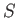is(1.29)

where the integral is taken over all outward directed elements of. Suppose, finally, that the property in question is created within the volumeat the rateper second. The conservation equation for the fluid property takes the form(1.30)

In other words, the rate of increase in the amount of the property contained withinis the difference between the creation rate of the property inside, and the rate at which the property is convected out ofby fluid flow. The previous conservation law can also be written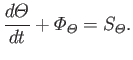(1.31)

Here,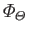is termed the flux of the property out of, whereasis called the net generation rate of the property within.Next: Mass Conservation Up: Mathematical Models of Fluid Previous: Viscosity
Richard Fitzpatrick 2016-03-31